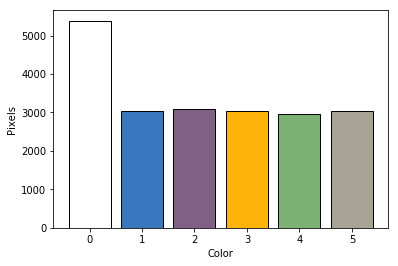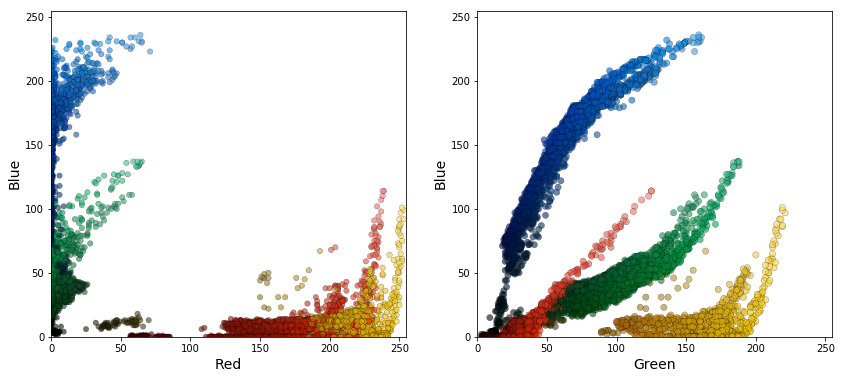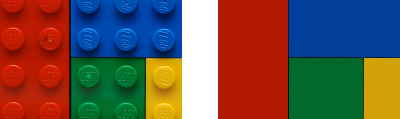This post deals with the question of how to determine the dominant colors in a given image, which is different from determining the average color of an image. We start with a simple case:This image illustrates one of the seaborn color palettes. If we extract the colors of the pixels in the image we can construct a histogram showing the number of pixels per unique color:From this histogram we now know the dominant colors in this very simple image. White is the dominant color because there is an invisible white frame encapsulating the five squares. The other five bars are not exactly the same height because the squares in the original image are not perfect squares. What if we consider a slightly more complicated image like the one below?In this case the color histogram becomes unreadable, which makes it impossible to draw any conclusions:These thousands of colors are caused by a large number of tiny variations due to shadows in the image. To determine the dominant colors in this image we need another approach: k-means clustering, which is considered a machine learning technique.

Excerpt from Wikipedia on k-means clustering:

k-means clustering aims to partition n observations into k clusters in which each observation belongs to the cluster with the nearest mean, serving as a prototype of the cluster. This results in a partitioning of the data space into Voronoi cells.

We apply k-means clustering to the data in the color histogram above to reduce the number of colors to a small, representative set: first, the colors are assigned to a cluster, second, we determine the average color within each cluster, which should result in a small number of representative, dominant colors.

Before applying the clustering algorithm we need to determine how to mathematically represent the colors. An obvious choice is the RGB (Red, Green, Blue) basis, which is widely used in programming, since all pixels in a modern monitor (computer, TV, phone) are essentially made of three smaller pixels in red, green and blue placed close together. The figure below shows two 2D plots of the color data in the RGB basis plotted first in B-R and second in B-G. Every point in the figure corresponds to, and is colored by, one of the 8460 unique colors present in the original image.A 3D scatter plot of the same data is shown below. Here, the size of each point is proportional to the number of pixels of that color in the original image.From these two figures it is already possible to determine four distinct clusters (and the k-means clustering algorithm will also work in this case). However, in my opinion, this basis does not seem intuitive. A more sensible basis for representing colors is HSV (Hue, Saturation, Value). A schematic of this basis is shown below (source).The figure below shows two ways of plotting the color data using the HSV basis. These plots correspond to looking at the HSV schematic above from the top.In the left part of the figure the H and S components are transformed to polar coordinates. In the right part the V component is included to make the dark shades meet in the center as in the HSV schematic above. From the left panel we see that if we choose the HSV basis we can distinguish the clusters by just considering the H and S components. This way we reduce the dimensionality (number of features) of the clustering problem from three to two. The block below explains in pseudo code how the color data is transformed to polar coordinates for the plots in the two figures above. The transformation is based on H, S and V all being numbers between zero and one.

``````Left:
x = S * cos(H * 2 * pi)
y = S * sin(H * 2 * pi)

Right:
x = V * S * cos(H * 2 * pi)
y = V * S * sin(H * 2 * pi)``````

The figure below shows a 3D representation of the right part of the figure just above.Having chosen the HSV basis for the color data, the dominant colors are determined via the following steps:

1. H and S values are transformed to polar coordinates as described above.
2. k-means clustering is performed on the transformed H and S values to partition all unique colors into four clusters.
3. The weighted average (based on number of pixels of each color in the original image) color within each cluster results in a set of dominant colors.

Below is an interactive plot of the color data as well as the dominant colors resulting from k-means clustering.

Finally, a comparison of the original image with an approximated version based on the dominant colors determined using k-means clustering.In this case the four dominant colors are able to produce a good approximation to the original image.

For those interested, I made a Python notebook with all the used code available on GitHub.